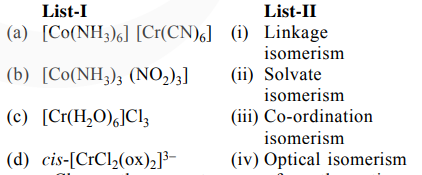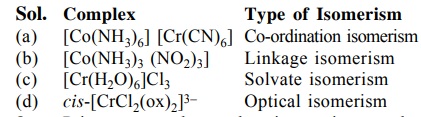# Match List-I with List-II :Question:

Match List-I with List-II :Choose the correct answer from the options given below :

1. (a)-(iii), (b)-(i), (c)-(ii), (d)-(iv)

2. (a)-(iv), (b)-(ii), (c)-(iii), (d)-(i)

3. $(\mathrm{a})-(\mathrm{ii}),(\mathrm{b})-(\mathrm{i}),(\mathrm{c})-(\mathrm{iii}),(\mathrm{d})-(\mathrm{iv})$

4. (a)-(i), (b)-(ii), (c)-(iii), (d)-(iv)

Correct Option: 1

Solution: